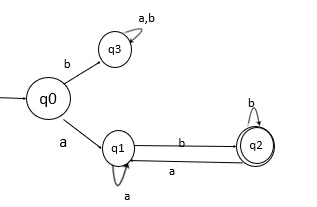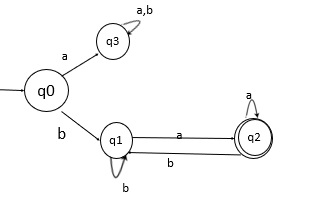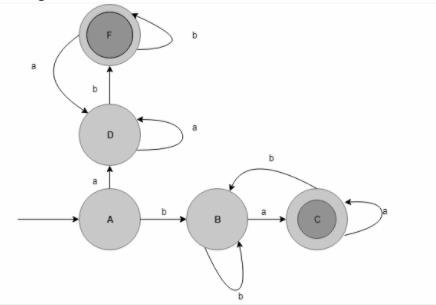# Explain Union process in DFA

The union process in the deterministic finite automata (DFA) is explained below −

If L1 and If L2 are two regular languages, their union L1 U L2 will also be regular.

## For example,

L1 = {an | n > O} and L2 = {bn | n > O}

L3 = L1 U L2 = {an U bn | n > O} is also regular.

## Problem

Design a DFA over an alphabet {a,b} where the start and end are of different symbols.

## Solution

There are two different types of languages are formed for a given condition −

• L1={ab,aab,abab,abb,…….}
• L1={ab,aab,abab,abb,…….}

Here,

• L1= starts with a and end with b
• L2= starts with b and ends with a

Therefore,

L=L1 U L2

Or

L=L1+L2

State transition diagram for L1

The state transition diagram for the language L1 is given below −The above DFA accepts all strings starting with a and ending with b.

Here,

• q0 is the initial state.
• q1 is an intermediate state.
• q2 is the final state.
• q3 is the dead state.

State transition diagram for L2

The state transition diagram for language L2 is as follows −The above DFA accepts all strings starting with b and ending with a.

Here,

• q0: Initial state.
• q1: Intermediate state.
• q2: Final state.

Now the union of L1 and L2 gives the final result of language which starts and ends with different elements.

The state transition diagram of L1 U L2  is as follows −Updated on: 15-Jun-2021

2K+ Views